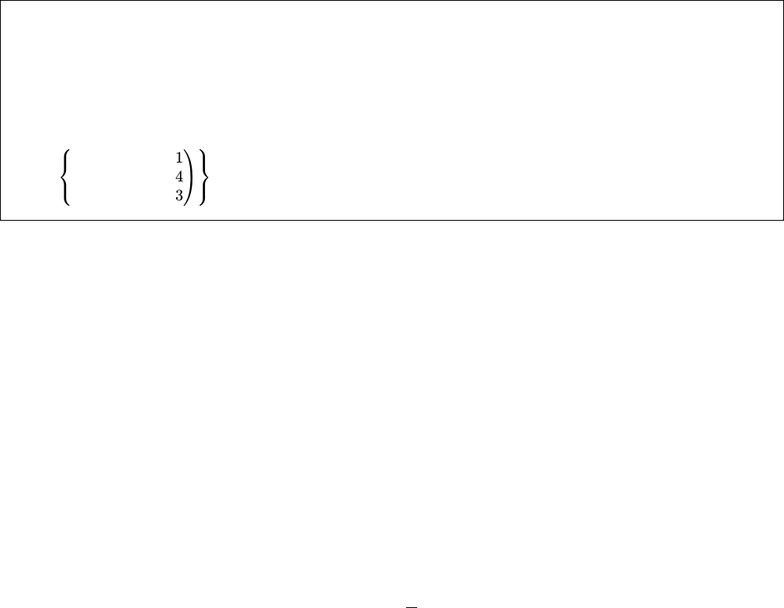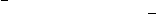Study Guides (400,000)
US (230,000)
CU-Boulder (1,000)
MATH (100)
All (7)
Midterm

# MATH 3001 Study Guide - Midterm Guide: Identity Matrix, Gaussian Elimination, Linear CombinationExam

Department
Mathematics
Course Code
MATH 3001
Professor
All
Study Guide
Midterm

This preview shows pages 1-2. to view the full 8 pages of the document.APPM 2360 Exam 2, Review 1 Solutions
Please do not think that the info on this page is something you should have memorized (except for
the ﬁrst list...that’s important). Rather, these facts may be helpful to use in problems, but are fairly
straightforward to prove (so understand them, but don’t go overboard in memorizing them).
The following are equivalent statements (meaning they are either all true or all false) for an n×nmatrix A.
Ais invertible (i.e. A1exists)
det(A)6= 0
Acan be row reduced to the identity matrix I
Ahas npivots
rank(A) = n
A~x =~
bhas a unique solution
A~x =~
0only has the trivial solution
Col(A) is a basis
Null(A) = n~
0o
The columns of Aas vectors are linearly independent
The columns of Aas vectors are a basis for Rn
The following facts regarding the inverse of a matrix apply so long as Aand Bare invertible.
A11=A
(kA)1=k1A1for k6= 0
AT1=A1T
(AB)1=A1B1
det A1=1
det(A)
The following facts regarding the transpose of a matrix apply for any matrices A, B and scalar c.
ATT=A
(A+B)T=AT+BT
(AB)T=BTAT
(cA)T=cAT
det AT= det(A)
If Ais a square matrix, then its eigenvalues are equal to the eigenvalues of its transpose.
The following facts regarding the determinant of a matrix apply for any square matrices Aand B.
If Aand Bare the same size, then det(AB) = det(A)·det(B)
If Ais n×n, then det(cA) = cndet(A)
If Ais upper or lower triangular, then det(A) = (a11)(a22)· · · (ann)
If any row or column of a matrix Aconsists of all zeros, then det(A) = 0
If any two columns of a matrix Aare identical (or can be written as a linear combination of other rows,

Only pages 1-2 are available for preview. Some parts have been intentionally blurred.Linear Independence of Vectors: Determine if the following collection of vectors in R3are linearly inde-
pendent. If the vectors are linearly dependent, ﬁnd a basis for the set of vectors
(a)
2
1
6
,
5
2
2
,
1
2
9
(b)
1
2
2
,
0
6
3
,
1
4
3
(a) If we let Abe the matrix with columns that are the vectors of this set, then we can row reduce it and gain
A=
251
122
629
R1
=R1R2
=
131
122
629
R2
=R1R2
=
1 3 1
0 1 1
6 2 9
R1
=R13R2
=
1 0 4
0 1 1
6 2 9
R3
=R36R1
=
1 0 4
0 1 1
0 2 15
R3
=R32R2
=
1 0 4
0 1 1
0 0 13
R3
=
1
13 R3
=
1 0 4
0 1 1
0 0 1
R2
=R2+R3
=
104
010
001
R1
=R14R3
=
100
010
001
Since Arow reduces to the identity matrix, we may say that Ais “row equivalent” to I. Thus, Ais
invertible. This implies that the columns of Awritten as vectors are linearly independent. So, the set of
vectors that was given are linearly independent.

Unlock to view full version

Only pages 1-2 are available for preview. Some parts have been intentionally blurred.(b) If we let Bbe the matrix with columns that are the vectors of this set, then we can row reduce it and gain
B=
101
264
233
R2
=R22R1
=
101
062
233
R3
=R32R1
=
101
062
031
R3
=R22R3
=
101
062
000
R2
=1
6R2
=
1 0 1
0 1 1
3
0 0 0
We see that we have a row of zeros in the third row. Clearly, there are two pivots columns (the ﬁrst
and second columns). This means that the column space of Bhas a basis
1
2
2
,
0
6
3
.
Since this is diﬀerent from Col(B), we know that Col(B) is not a basis. Therefore, Bis not invertible.
This implies that the columns of Bwritten as vectors are linearly dependent. So, the set of vectors that was
given are linearly dependent.